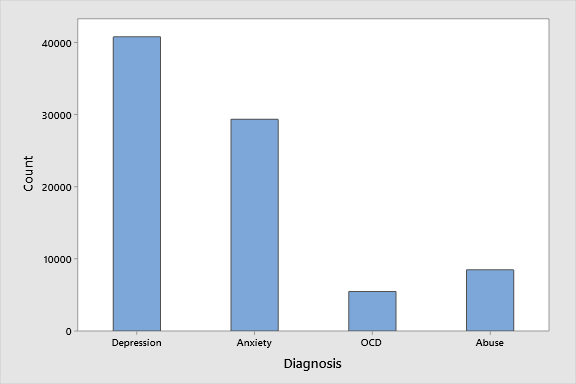# 1.2 - Summarizing Data Visually

1.2 - Summarizing Data Visually

## Summarizing Categorical Variables

Once the type of data, categorical or quantitative is identified, we can consider graphical representations of the data, which would be helpful for Maria to understand.

Frequency tables, pie charts, and bar charts are the most appropriate graphical displays for categorical variables. Below are a frequency table, a pie chart, and a bar graph for data concerning Mental Health Admission numbers.

Frequency Table
A table containing the counts of how often each category occurs.
 Diagnosis Count Percent Depression 40835 48.5% Anxiety 29388 34.9% OCD 5465 6.5% Abuse 8513 10.1% Total 84201 100.0%
Pie chart

Graphical representation for categorical data in which a circle is partitioned into “slices” on the basis of the proportions of each category.

##### Pitfalls

One of the pitfalls of a pie chart is that if the “slices” only represent percentages the reader does not know how many actual people fall in each category.

Bar Chart
Graphical representation for categorical data in which vertical (or sometimes horizontal) bars are used to depict the number of experimental units in each category; bars are separated by space.Note that in the bar chart, the categories of mental health diagnoses (bars) have white spaces in between them. The spaces between the bars signify that this is a categorical variable.

Pie charts tend to work best when there are only a few categories. If a variable has many categories, a pie chart may be more difficult to read. In those cases, a frequency table or bar chart may be more appropriate.

##### Pitfalls

While bar charts can be presented as either percentages (in which case they are referred to as relative frequency charts) or counts, the differences among the heights of the bars are often assumed to be different, even when they are not.

## Summarizing Quantitative Variables

But what of variables that are quantitative such as math SAT or percentage taking the SAT? For these variables we should use histograms or boxplots. Histograms differ from bar graphs in that they represent frequencies by area and not height. A good display will help to summarize a distribution by reporting the center, spread, and shape for that variable.

For now, the goal is to summarize the distribution or pattern of variation of a single quantitative variable.

Histogram
Histograms are graphical displays that can be used with one quantitative variable. In these plots the horizontal axis represents the values of the variable and the height of the bar represents how many observations are equal to the particular value.

From the histogram of children’s heights below, Maria can see that about 10 children have a height equal to “60”.##### Pitfalls

People frequently confuse bar charts and histograms. The first test should be to identify what kind of data you are charting (or what kind of data was charted), quantitative or categorical. Another hint will be that the x-axis of the histogram will contain labels that reflect a quantitative variable, bar charts will have an x-axis that contains category labels, generally not numbers.

To draw a histogram by hand we would:

1. Divide the range of data (range is from the smallest to largest value within the data for the variable of interest) into classes of equal width.
2. Count the number of observations in each class.
3. Draw the histogram using the horizontal axis as the range of the data values and the vertical axis for the counts within the class.

## Choosing the appropriate display

When selecting a visual display for your data you should first determine how many variables you are going to display and whether they are categorical or quantitative. Then, you should think about what you are trying to communicate. Each visual display has its own strengths and weaknesses. When first starting out, you may need to make a few different types of displays to determine which best communicates your data.

  Link ↥ Has Tooltip/Popover Toggleable Visibility